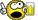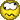# class to handle very large number of significant figuresClassicListThreaded6 messagesOpen this post in threaded view
|

## class to handle very large number of significant figures

 As part of project 9 is was thinking of doing the above. What I'm wondering is if there's a mistake in my thinking concerning design (and whether it's possible to implement at all) So the idea is that you'd have something like:  var NumBer x let x = -273235837434000000057345374530.000030040340050345030523462 (Am implementing in this way  let x=NumBer.new("-273235837434000000057345374530.000030040340050345030523462"); and transferring the string to a number array) So far so good (ie I can convert a long strings (at least 50 chars) into number arrays appropriately aligned).. . For addition and subtraction the decimal points have to be aligned (is that really true ? I guess so) but not so for multiplication or division? In order to speed things up, rather than storing each digit in an array element, I'm storing 4 digits (max 9999) so once i've got my decimal points aligned it should be fairly trivial to add or subtract. eg 27 3235 8374 3400 0000 0573 4537 4530.0000 3004 0340 0503 4503 0523 462       plus                                 3457 3456 9834 5739.2346 3997 1100 0001 2200 easy. My worry is multiplication etc. (I wrote a similar class in C++, but it's not very well written and it's well over 600 lines, eek) Obvs I'll have to implement Equals and maybe Less Than as well. And the division ! Before I go a 'waste' a few weeks on this, does anyone see any holes in my thinking etc etc. Any input much appreciated even if you think i'm bonkers.Then if I can get it to work, use that class to do some mandlebrot drawing...Thanks in advance
Open this post in threaded view
|

## Re: class to handle very large number of significant figures

 There are 2 popular ways of representing decimal numbers: fixed point and floating point representation. It looks to me, like you are trying to implement something like arbitrary precision fixed point. Am I right? If so, you'll still have to provide the precision. Consider this: let one = Number.new("1"); let three = Number.new("3"); let one_third = one.dev(three); This will create the number 0.33333333...., which you cannot hope to fully represent in any amount of memory in decimal form. And there are numbers, which cannot be represented fully in any form, like pi. So you'll need to somehow specify the precision of the result. Lozminda wrote In order to speed things up, rather than storing each digit in an array element, I'm storing 4 digits (max 9999) You are thinking in decimal digits. An int in Jack is 16-bit, which means it can contain numbers from -32768 to 32767, so you'll have no problems with addition and subtraction. You're loosing some space (you only use 10000 values from the 65536 possible in a 16-bit word). Multiplication would create additional problems though. 9,999 * 9,999 = 99,980,001, which Jack won't be able to multiply directly. You may consider using binary arithmetic instead and treat the array as one large binary number. Another consideration is, that in Jack the string constants are actually objects allocated on the heap. So the above code allocates two strings and does not free the memory afterwards, meaning it has memory leaks.
Open this post in threaded view
|

## Re: class to handle very large number of significant figures

 Hi, and thanks very much for your reply.. It looks to me, like you are trying to implement something like arbitrary precision fixed point. Am I right?Yes. Indeed i have thought about recuring decimals, and would have a method SetDecimalLimit() or some such, to stop me disappearing of to infinity..... You are thinking in decimal digits. An int in Jack is 16-bit, which means it can contain numbers from -32768 to 32767, so you'll have no problems with addition and subtraction. You're loosing some space (you only use 10000 values from the 65536 possible in a 16-bit word). Multiplication would create additional problems though. 9,999 * 9,999 = 99,980,001, which Jack won't be able to multiply directly. You may consider using binary arithmetic instead and treat the array as one large binary number.I used 9999, because 9999 + 9999 won't overflow anything, and to keep it a bit easier for me, and i'm not sure how i'd implement it other wise. Moving to binary. I guess i'd do everything in binary and then only convert to decimal if i wanted some kind of output.. I've used a string for input eg let x = NumBer.new("3409857340597250987609869463409687209872096723409") because I can't think of a way to get the large number 'In' to x. It need to be in some initial format that jack will accept. If i did the above not as a string then i'll get an overflow... let x = NumBer.new(3409857340597250987609869463409687209872096723409) surely for my input to the constructor I need to use a type that recognisable to the jack API, and the only one that can be arbitrarily large is String.. I'm really really happy to be wrong about the above, coz it'll solve my memory leak (another question i had hanging around in my head) and I imagine String to be relatively slow. Concerning the memory leak, I wasn't sure whether String passed as a parameter would then be destroyed when the function returned.. ie in pseudo code:- function void Bob (String SomeInput) {    var Variable    Do some stuff with Variable and SomeInput                     // So in jack I have to dispose of SomeInput before return. If it was some user type                     // I was passing as a param (eg Bob (MyClass SomeInput) ) i'd have to dispose of that too I guess?                     // Even though function is a void ?                    // The correct way would be SomeInput.destroy() (pseudo code) then:   return } Thanks again that was really helpful ! Lozminda   PS I guess poking (and peeking) 'everything' will give me better performance (as well as working in binary).. ?
Open this post in threaded view
|

## Re: class to handle very large number of significant figures

 Lozminda wrote I've used a string for input eg let x = NumBer.new("3409857340597250987609869463409687209872096723409") because I can't think of a way to get the large number 'In' to x. It need to be in some initial format that jack will accept. If i did the above not as a string then i'll get an overflow... let x = NumBer.new(3409857340597250987609869463409687209872096723409) surely for my input to the constructor I need to use a type that recognisable to the jack API, and the only one that can be arbitrarily large is String.. Sorry, I didn't make it clear. String is the best option. I was just pointing out, that one has to be careful with how Jack compiler handles String constants. This is a problem even with built-in methods, like print. When you implement the Jack compiler in the next chapters, you'll see that when it sees a string constant it creates a new String object by actually calling String.new. But it doesn't dispose it, because it cannot know when it's safe to do so. Lozminda wrote Concerning the memory leak, I wasn't sure whether String passed as a parameter would then be destroyed when the function returned.. ie in pseudo code:- function void Bob (String SomeInput) {    var Variable    Do some stuff with Variable and SomeInput                     // So in jack I have to dispose of SomeInput before return. If it was some user type                     // I was passing as a param (eg Bob (MyClass SomeInput) ) i'd have to dispose of that too I guess?                     // Even though function is a void ?                    // The correct way would be SomeInput.destroy() (pseudo code) then:   return } In languages like Jack and C it is very important to track who "owns" the object. That is, who is responsible for the cleaning up. And there is no one right answer to this. You can provide Consider this code: var NumBer n1, n2; var String s; let s = "123.456"; let n1 = NumBer.new(s); let n2 = NumBer.new("789.0123"); do Screen.print(s); If new disposes the parameter, then  you'll have a problem in the last line. If it doesn't, you'll have a memory leak. What you can do is to provide two constructors, one which disposes the parameter and one which doesn't. The former is the more general constructor, and the latter is there for convenience. Lozminda wrote PS I guess poking (and peeking) 'everything' will give me better performance (as well as working in binary).. ? Directly peeking and poking in memory is generally a bad idea. Higher level languages are there to provide abstractions, which one can use to write code more easily. For various reasons Jack cuts a lot of corners, so its abstractions are more leaky than what most real-world languages actually do. But you still should avoid going around them most of the time. Plus, I don't really see how they are faster in this case. Peek and poke themselves are implemented as array access.